Test: Congruence Criteria- SSS And RHS

# Test: Congruence Criteria- SSS And RHS

Test Description

## 10 Questions MCQ Test Mathematics (Maths) Class 9 | Test: Congruence Criteria- SSS And RHS

Test: Congruence Criteria- SSS And RHS for Class 9 2022 is part of Mathematics (Maths) Class 9 preparation. The Test: Congruence Criteria- SSS And RHS questions and answers have been prepared according to the Class 9 exam syllabus.The Test: Congruence Criteria- SSS And RHS MCQs are made for Class 9 2022 Exam. Find important definitions, questions, notes, meanings, examples, exercises, MCQs and online tests for Test: Congruence Criteria- SSS And RHS below.
Solutions of Test: Congruence Criteria- SSS And RHS questions in English are available as part of our Mathematics (Maths) Class 9 for Class 9 & Test: Congruence Criteria- SSS And RHS solutions in Hindi for Mathematics (Maths) Class 9 course. Download more important topics, notes, lectures and mock test series for Class 9 Exam by signing up for free. Attempt Test: Congruence Criteria- SSS And RHS | 10 questions in 10 minutes | Mock test for Class 9 preparation | Free important questions MCQ to study Mathematics (Maths) Class 9 for Class 9 Exam | Download free PDF with solutions
 1 Crore+ students have signed up on EduRev. Have you?
Test: Congruence Criteria- SSS And RHS - Question 1

### In isosceles ΔPQR, PQ = PR, M is the mid point of QR. LM ⊥ PQ, MN ⊥ PR. By which criterion of congruency is ΔQLM 0 ≅ ΔMNR.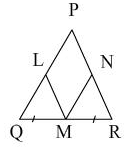Test: Congruence Criteria- SSS And RHS - Question 2

### ABCD is a parallelogram, if the two diagonals are equal, then by what criterion are the triangles ABD and ABC congruent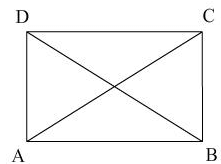Test: Congruence Criteria- SSS And RHS - Question 3

### In ΔABC and ΔPBC, AB = BP and AC = PC. Can you say whether the triangles are congruent to each other or not:

Test: Congruence Criteria- SSS And RHS - Question 4

In the given figure, AB = PQ, BC = QR and the median AD is equal to the median PM of the other triangle PQR, then ΔABD is congruent  ΔPQM by the criterion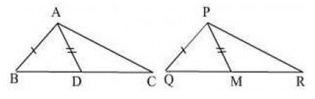Test: Congruence Criteria- SSS And RHS - Question 5

Two equilateral triangles are congruent when:

Test: Congruence Criteria- SSS And RHS - Question 6

In the following figure, if PQR ≅ ABC, then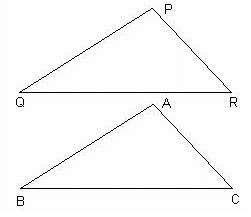Test: Congruence Criteria- SSS And RHS - Question 7

Choose the correct statement

Test: Congruence Criteria- SSS And RHS - Question 8

In ΔABC and PQR, AB = QR, BC = RP, AC = QP. So,

Detailed Solution for Test: Congruence Criteria- SSS And RHS - Question 8 Option b is correct because when we write the names of triangle we write according to the corresponding parts of one triangle to otherfor eg. in ∆abc and ∆qrp we have ab = qr which also corresponds to the corresponding parts of congurent triangles property
Test: Congruence Criteria- SSS And RHS - Question 9

In the following figure, PT is the bisector of ___________.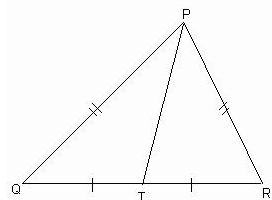Test: Congruence Criteria- SSS And RHS - Question 10

PQRS is a parallelogram, if the two diagonals are equal, then the measure of PQR is:

## Mathematics (Maths) Class 9

84 videos|352 docs|109 tests
 Use Code STAYHOME200 and get INR 200 additional OFF Use Coupon Code
Information about Test: Congruence Criteria- SSS And RHS Page
In this test you can find the Exam questions for Test: Congruence Criteria- SSS And RHS solved & explained in the simplest way possible. Besides giving Questions and answers for Test: Congruence Criteria- SSS And RHS , EduRev gives you an ample number of Online tests for practice

## Mathematics (Maths) Class 9

84 videos|352 docs|109 tests